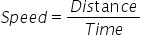Mathematics
Easy

Question

# An aeroplane flies with a constant speed of 600 miles per 5 hours. If it travels at the same rate, distance travelled in 8 hours by the plane is ________________.

## 960900850750Hint:

## The correct answer is: 960

### The given values of distance and time isDistance = 600 milesTime = 5 hoursTo solve the equation, we will find speed. We will find the speed by taking the ratio of distance to time. As speed is constant, we will use this to find the value of the distance travelled in 8 hours.Let’s see the formulaLet’s use the value of speed to find the second distance..We will rearrange the formula for distance. We will multiply the L.H.S and R.H.S of the equation by time.Speed × Time = DistanceDistance = Speed × Time= 120 × 8= 960 milesTherefore, the distance travelled by the areoplane in 8 hours is 960 miles.

The other method is to take the ratios and directly equate them. As the rate is constant, so the ratios will be constant. So we will consider the quantity we have to find as x and then equate the ratios. It will be a shortcut.

### Related Questions to study#### With Turito Foundation.#### Get an Expert Advice From Turito.